International
Tables for
Crystallography
Volume C
Mathematical, physical and chemical tables
Edited by E. Prince

International Tables for Crystallography (2006). Vol. C, ch. 2.6, pp. 111-112

## Section 2.6.2.7.7. Triple isotropic replacement

R. Mayb

#### 2.6.2.7.7. Triple isotropic replacement

| top | pdf |

An elegant way of determining the structure of a component inside a molecular complex has been proposed by Pavlov & Serdyuk (1987). It is based on measuring the scattering curves from three preparations. Two contain the complex to be studied at two different levels of labelling,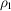and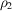, and are mixed together to yield sample 1, the third contains the complex at an intermediate level of labelling,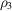(sample 2). If the condition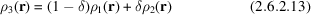is satisfied by δ, the relative concentration of particle 2 in sample 1, then the difference between the scattering from the two samples only contains contributions from the single component. Additionally, the contributions from contamination, aggregation, and interparticle effects are suppressed provided that they are the same in the three samples, i.e. independent of the partial-deuteration states.

In the case of small complexes, δ can be obtained by measuring the scattering curves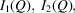and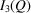of the three particles as a function of contrast and by plotting the differences of the zero-angle scattering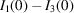and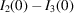versus δ. The two curves intercept at the correct ratio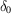.

The method, which can be considered as a special case of a systematic inverse contrast variation of a selected component, holds if the concentrations, the complex occupations, and the aggregation behaviour of the three particles are identical. Mathematically, the difference curve is independent of the contrast of the rest of the complex with respect to the solvent. In practice, it would be wise to follow the same considerations as with triangulation.

### References

Pavlov, M. Yu. & Serdyuk, I. N. (1987). Three-isotropic substitutions method in small-angle neutron scattering. J. Appl. Cryst. 20, 105–110.Google Scholar